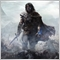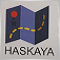# Calculate daily maximum loss216

HI good day everyone please help me i am tryna say in this logic to stop loss at a certain equity loss level see my code i dont know what im doing wrong here So in My code i have calculated daily trades maximum profit so i called this function to use for maximum daily profit it worked but when i invert logic for loss to close all trades when certain loss has been incurred already Please help
```//+------------------------------------------------------------------+
//|                                                     DMF Real.mq4 |
//|                 Copyright 2021, ITace Inc. (Marve)Software Corp. |
//|                                             https://www.ITace.com |
//+------------------------------------------------------------------+
#property version   "1.00"
#property strict
input double SL= 0.5;
input double TP= 0.5;
input double TND= 50;
input double LotSize= 0.2;
input double SLS= 0.2;
input double SSL=1;
input double STP=0.5;
input double CLS=0.2;
input double CTP=0.5;
input double CSL=0.5;
input double ElapsedSECONDS = 1*60;
extern double MaxDailyProfit = 200;
extern double MaxDailyLoss = 200;
void OnTick()
{
double iHi = iHigh(Symbol(), PERIOD_M1, 0);
double iLo = iLow(Symbol(), PERIOD_M1, 0);
int ticket0, ticket2, ticket1,OrdrTotal = OrdersTotal();
bool Done4Today = Calc_Today_Closed_Profits(_Symbol)+Calc_OpenOrders_Profits(_Symbol)>MaxDailyProfit;
bool close4loss = Calc_Today_Closed_Profits(_Symbol)+Calc_OpenOrders_Profits(_Symbol)<MaxDailyLoss;
if(Done4Today==true)CloseAllOpenPoss(_Symbol);
if(Done4Today==false && OrdrTotal<1 && validTime==true)
{
ticket0=OrderSend(Symbol(),OP_SELL, LotSize, NormalizeDouble(bid,Digits),0,NormalizeDouble(bid+SL,Digits),NormalizeDouble(bid-TP,Digits),"Order0",2000,0,clrRed);
}
{
if(OrderMagicNumber()==2000 && OrderSymbol()==_Symbol)
{
long ElapsSec = MathMin(MinOrdOpElaps(_Symbol),MinOrdClElaps(_Symbol));
bool LastClosedByLoss = LastOrdClosedByLoss(_Symbol);
if(LastClosedByLoss)Print("LastClosedByLoss!!!");
{
{
Print(" OrderSend... "," ElapsSec:",ElapsSec,"  ElapsedSECONDS:",ElapsedSECONDS);
ticket1=OrderSend(_Symbol,OP_SELL,CLS, NormalizeDouble(bid,Digits),0,NormalizeDouble(bid+CSL,Digits),NormalizeDouble(bid-CTP,Digits),"Order1",2000,0,clrRed);
}
else
{
//Print(" waiting4Order1... ElapsSec:",ElapsSec);
}
}
if((OrderType()==OP_SELL) &&validTime==true)
{
{
Print(" OrderSend... "," ElapsSec:",ElapsSec,"  ElapsedSECONDS:",ElapsedSECONDS);
}
else
{
//Print(" waiting4Order1... ElapsSec:",ElapsSec);
}
}
}

}
}
//+------------------------------------------------------------------+
long MinOrdOpElaps(string symb)
{
long dif=1e+16;
for(int o=0; o<OrdersTotal(); o++)
{
{
if(OrderSymbol()==symb)
{
if(TimeCurrent()-OrderOpenTime()<dif)
dif=TimeCurrent()-OrderOpenTime();
}
}
}
return(dif);
}
//+------------------------------------------------------------------+
long MinOrdClElaps(string symb)
{
long dif=1e+16;
int o = OrdersHistoryTotal(),a = OrdersHistoryTotal() - 10,b = OrdersHistoryTotal();
for(o = a; o < b ; o++)
{
if(OrderSelect(o,SELECT_BY_POS,MODE_HISTORY))
{
if(OrderSymbol()==symb)
{
if(TimeCurrent()-OrderOpenTime() <dif)
dif=TimeCurrent()-OrderOpenTime();
if(TimeCurrent()-OrderCloseTime()<dif)
dif=TimeCurrent()-OrderCloseTime();
}
}
}
return(dif);
}
//+------------------------------------------------------------------+
bool LastOrdClosedByLoss(string symb)
{
bool ClosedByLoss=false;
static datetime lastOrderCloseTime;
int o = OrdersHistoryTotal(),a = OrdersHistoryTotal() - 10,b = OrdersHistoryTotal();
for(o = a; o < b ; o++)
{
if(OrderSelect(OrdersHistoryTotal()-1,SELECT_BY_POS,MODE_HISTORY))
{
if(OrderSymbol()==symb &&  OrderCloseTime()> lastOrderCloseTime)
{
lastOrderCloseTime = OrderCloseTime();
ClosedByLoss = MathAbs(OrderClosePrice()-OrderStopLoss())  < MathAbs(OrderClosePrice()-OrderTakeProfit()) ;
}
}
}
return(ClosedByLoss);
}
//+------------------------------------------------------------------+
#define HR2400 86400
#define SECONDS uint
SECONDS    time(datetime when=0) {return SECONDS(when == 0 ? TimeCurrent() : when) % HR2400;               }
//+------------------------------------------------------------------+
//|                                                                  |
//+------------------------------------------------------------------+
datetime   date(datetime when=0) {return datetime((when == 0 ? TimeCurrent() : when) - time(when));      }
bool isValidTime(SECONDS t0, SECONDS t1, datetime when=0) {SECONDS now = time(when); return t0 < t1 ? t0 <= now && now < t1  : !isValidTime(t1, t0, when);}
//+------------------------------------------------------------------+
//|                                                                  |
//+------------------------------------------------------------------+
{
string res[];
StringSplit(str,StringGetCharacter("-",0),res);
string T0=res,T1=res;
int H0,M0,H1,M1;
ArrayFree(res);
StringSplit(T0,StringGetCharacter(":",0),res);
H0=StrToInteger(res);
M0=StrToInteger(res);
t0=H0*60*60+M0*60;
ArrayFree(res);
StringSplit(T1,StringGetCharacter(":",0),res);
H1=StrToInteger(res);
M1=StrToInteger(res);
t1=H1*60*60+M1*60;
}
//+------------------------------------------------------------------+
//|                                                                  |
//+------------------------------------------------------------------+
{
int t0,t1;
return(isValidTime(t0,t1));
}
//+------------------------------------------------------------------+
double Calc_Today_Closed_Profits(string symb)
{
double PNL= 0; for( int cnt = 0; cnt <= OrdersHistoryTotal(); cnt++)
if ( OrderSelect( cnt, SELECT_BY_POS, MODE_HISTORY ) )
if( OrderSymbol()==symb && OrderType() < 2 && iTime(symb, PERIOD_D1,0) <= OrderCloseTime() )
PNL += OrderProfit() + OrderSwap() + OrderCommission();
return(PNL);
}
//+------------------------------------------------------------------+
double Calc_OpenOrders_Profits(string symb)
{
double profit=0;
for(int o=0; o<OrdersTotal(); o++)
{
{
if(OrderSymbol()==symb)
{
profit+=OrderProfit();
}
}
}
return(profit);
}
//+------------------------------------------------------------------+
void CloseAllOpenPoss(string symb)
{
int total = OrdersTotal();
for(int i=total-1;i>=0;i--)
{
if(OrderSelect(i,SELECT_BY_POS) && OrderSymbol()==symb)
{
bool result = false;
switch(OrderType())
{
case OP_BUY       : result = OrderClose( OrderTicket(), OrderLots(), MarketInfo(OrderSymbol(), MODE_BID), 5, Red );break;
case OP_SELL      : result = OrderClose( OrderTicket(), OrderLots(), MarketInfo(OrderSymbol(), MODE_ASK), 5, Red );break;
}
if(result == false)Sleep(3000);
}
}
}
{
double free_margin=AccountFreeMarginCheck(symb,type,lots);
if(free_margin> 0)
return(true);
return (false);

}```712

Why don't you use any comments?

It makes it so much simpler for other persons to read and understand your code.

Also for you, if f.e. you have to change something on your code a year later.

I really wanted to help you but I have not the time to read the whole whole code and try to understand what you have coded...

How and Why to Comment C and C++ Code - Cprogramming.com
• www.cprogramming.com
Learn why to comment your C and C++ code--and how you should do it.216

Lukas Roth #:

Why don't you use any comments?

It makes it so much simpler for other persons to read and understand your code.

Also for you, if f.e. you have to change something on your code a year later.

I really wanted to help you but I have not the time to read the whole whole code and try to understand what you have coded...`extern double MaxDailyLoss = -200;`